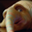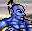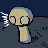# The miracles that matter

Wednesday, 12 February, Year 6 d.Tr. | Author: Mircea Popescu

Let's work our minds together, it'll be fun. So :

Part I.

Let's consider the set of all the cardinals of definite setsi, we'll call it the set of natural numbers and note it ℕ.

We can now define the function addition, which takes two elements out of ℕ and adds them together. For instance :

2 + 4 = 6

Addition happens to be a commutative operation, which means it yields the same result irrespective of the order of the two elements :

4 + 2 = 6

Since in the lovely field of mathematics there exists no entropy and thus no timeii any function can be reversed, which is to say we can define the function subtraction, which given a pair of numbers yields that third number which at some point in the indefinite past was added to the second to yield the first. Like so :

6 - 4 = 2

2 being that number which was sometime added to 4 in order to yield 6. Unlike addition, however, subtraction is not commutative. That is to say,

4 - 6 = -2

-2 is clearly not 2, and what's worse, -2 is not actually in ℕ, as there may exist an empty bag in which if you put two eggs you'll get a bag with two eggs in it, and there may also exist a bag with two eggs in it from which you can thus extract two eggs rendering it empty. However there exists no bag in which you can insert two eggs in order to thus render it empty, nor an empty bag from which you could pull forth two eggsiii. Which means that by logically extending the original addition function through constructing its reverse, we've also forced an expansion of our original set of cardinals. And so ℤ is borniv.

ℤ is simply ℕ with its evil mirror -ℕ, which is to say 1 and -1, 2 and -2, 56 and -56 and so on. Now on this ℤ we can define the function multiplication, which takes two elements and multiplies them together. Like so :

2 × 4 = 8

Much like the earlier addition, multiplication is also commutative, which is to say

4 × 2 = 8

And much like in the case of addition an inverse could be constructed, which is to say a function which given two numbers would find the third which multiplied with the second would yield the first. Like this :

8 ÷ 4 = 2

This would be called division, and much like subtraction, it is not commutative. To wit :

4 ÷ 8 = 1/2

1/2, or 0.5 as it is otherwise known, is a fraction, which is to say not an integer, which is to say not Zahlen and thus not in ℤ. So yet again we've ended up extending our original set of "numbers" to include even more types of numbers to satisfy the ever growing demands of our synthetic constructs which at the time seemed logical and down the road ended up spelling trouble. And thus ℚ is born.v

ℚ is the set of rational numbers, which is to say all natural ℕs, its evil mirror the un-natural -ℕ as well as all un-integers ie the fractions, much to teenagers' delight.

ℚ of course allows the following abstraction be introduced, that the function power is defined, which simply multiplies its first element with itself as many times as the second counts. This function can well be defined on ℤ, because :

4 2 = 16

whereas

4 -2 = 1/16vi

So now we're stuck with a function defined on ℚ with elements from ℤ ? What nonsese is this, seriously. It should properly be expanded so it takes elements from ℚ and gives results in ℚ, if indeed ℚ is to hold its rank of truely and definitively the last and ultimate all-numbers concentration camp. (Javohl!)

What could a fraction possibly mean should it happen in the 2nd spot however ? Quite obviously... the reverse function of this raising to the power. Thus

4 1/2 = the square root of 4 = that number which multiplied with itself twice yields 4.

And now... suddenly we've lost commutativity, because

2 1/4 = 2 and god help us.

God help us, because the square root of 2 is not a fraction, nor could it be expressed as a finite decimal notation. It's only 1.414213562 because your computer runs out of decimals there, but with a better one you could see it's really endless.

So now it seems we've managed to expand ℚ again. Fine, but let this be for the last time : we'll call it ℝ for real numbers and let it include nonsense like the square root of two, with the strong implication behind the name being, of course, that we are finally done : really and definitively 4ever&ever, these are the numbers and we're done with the nonsense.

Except... what do you suppose would be the square root of -1 ? Clearly a negative multiplied with itself could only yield a positive, and thus the square root of -1 could never yield a number, even in ℝ. Which means ℝ just got expanded again, we'll call the √-1 beauty i - short for imaginary, 'cause by now we've definitely run ourselves outside of reality - and the new set ℂ, for complex, because well... it's complicated.

ℂ will thus include all natural and all unnatural numbers, as well as the uninteger, irrational and imaginary. All ℂ numbers will be noted as a+ib, where a is the real part and ib the imaginary part, so that all ℝ numbers are simply ℂ numbers with a convenient b = 0 tail.

And now we're done, right ? Wrong, of course. The reason we're not done is expressed by Godel, we'll never be done, we can not be done. We can't ever have a set of numbers and a set of operations so that we include everything. Just how it goes.

This completes the first part of our miracle.

Part II.

Every circle also has a circumference.

The ratio of a circle's radius and a circle's circumference will always be π, which is an irrational number, much like the square root of 2.

This completes the 2nd part of our miracle.

Part III.

Suppose you have a Bitcoin on deposit somewhere, with a merchant of spotless reputation and infinite credibility, who pays you 100% per year. If the interest is credited at the end of the year, clearly after one year you will have two Bitcoins. What happens however if the interest is computed more often ?

If you get the interest calculated each 6 months, you will end up with 50% and 50% yielding 2.25 Bitcoin at the end of the year. If if is calculated each quarter you will end up with 2.44140625 Bitcoin.

And if it is calculated every millionth of a millionth of a millionth of a ... microsecond ? Why, you will end up with e Bitcoins. That's right, e, Euler's constant, it's the limit of f(x) = (1 + 1/x)x when x goes to infinity. An irrational number, just like π and i.

This completes the third and last part of our miracle, we are now ready to proceed. Behold :

# e π × i = -1

That's right baby : the piece-of-shit tail-of-the-devil problem-number we ended up straddled with while doing set theory, the essence of the circle and the essence of usury all come together, as if by magic, into one breathtakingly simple formula.

Why is this ? Why should this be ? What in the world and all the stars could these three things possibly have to do with each other ? And nevertheles... there they are.

So now : this is why educated gentlemen such as yourselves have no need, no use and no interest for pedestrian nonsense a la "intelligent design", and this is why we don't go to church, that wanna-be thought simulacrum built entirely in the manner of a ten year old wearing his father's pistol belt.

We don't need to, we got all the miracles we could possibly need right here. Enjoy!

———
1. The particular form of the definition is important for reasons. The meaning is as follows : any collection of anything is a set. A definite set is any collection which you could - in principle - produce for my inspection. In this sense the fingers on your hand are a definite set of fingers, the pockets on your jacket a definite set of pockets and the stars on the sky a definite set of stars, whereas your future jobs aren't a definite set of jobs, all the potatoes you could eat aren't a definite set of potatoes and so on. A cardinal is simply the count, for instance the cardinal of the set of the fingers on your hand is - in most cases - five. []
2. Because time is strictly the direction in which entropy flows, much like "down" is, as far as rubber ducklings in a tub are concerned, the direction in which the water sucks them. []
3. Ah, if only, how popular frittata'd be. []
4. ℤ stads for Zahlen, which in German means number, because Frege and the gang did a lot of work on the topic. []
5. It so happens that while ℕ and ℤ both have the same cardinal, which is to say contain the same count of elements, ℝ is actually larger. This is a topic of much advanced studying we won't really be covering here. []
6. This is why your height, say 187 centimeters, could be expressed as 1.86 × 10-3 kilometers just as well as 1.86 × 103 milimeters. []
Category: Trilenciclopedia

14 Responses

1.1
Stanislav DatskovskiyWednesday, 12 February 2014

This tale is sadly incomplete without a mention of the legendary drowned Pythagorean.

2.2
Mircea Popescu
Wednesday, 12 February 2014

As per Godel's express orders.

3.3
Anonimosław
Wednesday, 12 February 2014

Q larger? What kind of larger do you mean?

4.4
Mircea Popescu
Wednesday, 12 February 2014

It's like this :

If you take every odd natural number and associate it a negative integer, and then take every even natural number and associate it a positive integer, you will have created a one-to-one association between natural numbers and integers, which means the two sets are equal sizes.

If you try to enumerate fractional numbers so that they may be associated with natural numbers, for any subset of that enumeration a fractional number can be constructed which differs from each of the numbers on the list on a different decimal position (in the end you need as many decimal positions as the cardinal of the elected set). This means the natural numbers and the fractional numbers won't be placed in such a one-to-one association, and this means the natural numbers are fewer.

5.5
Anonimosław
Wednesday, 12 February 2014

Isn't your explanation for real numbers? I could totally fit whole ZxN into N even with some room left (up to aleph zero of unused N elements) and rationals are subset of ZxN. Sorry for poor formatting. I ne habla LaTeX.

6.6
Mircea Popescu
Wednesday, 12 February 2014

Aw shit we were discussing Q not R. Eh what do you want from me, it's been a while since 5th grade.

7.7
spykedWednesday, 19 March 2014

To expand, we could define cartesian products of sets using sets (theoretically we could define "sums" of sets too, but let's not go that far) and have something like A x B denoting all the ordered pairs (a, b), where a and b are elements of A and B respectively.

Then we can show that ℂ and ℝ x ℝ are correspondent to each other, or that well, they're isomorphic, but then we'd have to define isomorphisms; which is also true for ℚ and ℤ x ℤ, by the way (and that can be used to show that ℚ is countable).

But pairs are more important than that, since we can use them to define relations, which we can then use to define functions. And suddenly mathematics don't look that difficult when we start from these basic building blocks. :)

8.8
Mircea Popescu
Wednesday, 19 March 2014

This is quite so.

1. [...] "The miracles that matter", Mircea Popescu gives a beautiful description of numbers, starting from set-theoretical constructs [...]

2. [...] Which in any civilized world everywhere and everywhen means any intellectual, period. This is Leonardo's most important lesson: you're not human unless you're willing to understand the important things that your lazy reptilian brain otherwise refuses to, i.e. the miracles that matter. ↩ [...]

3. [...] I can do so at any level and to any degree of complexity you have the time and intelligence for. Like so. Should you require a random nigger to introduce his nonsense to you, he'll sit there paralyzed [...]

4. [...] i and n are natural numbers (positive integers and 0) ; the ai series is however rational, not real. And X can be anything. So you'd have to prove that given a certain n (the thing's "class" so to [...]

5. [...] reason : the odds are there that the constant's actually a natural constant, one of the dozen or so magic numbers of the universe. But if the author's inept, the odds are much better that the constant's actually just a creation [...]

6. [...] ci ca sa fie 3. diana_coman da mircea_popescu e numar magic, ca si cum ar vrea acuma sa fie alt e. diana_coman bun atunci; restul ca si format imi pare ok, nu vad deocamdata probleme [...]

»
If this is your first comment, it will wait to be approved. This usually takes a few hours. Subsequent comments are not delayed.